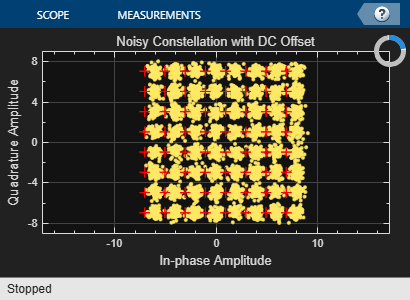# DC Blocker

Block DC component

## Library

Signal Operations

`dspsigops`

•## Description

The DC Blocker block removes the DC component of the input signal.

## Dialog Box

The DC Blocker dialog box changes based on how the DC offset is estimated. The dialog box for the `IIR` method is shown below.The dialog box for the `FIR` method is shown below.The dialog box for the `CIC` method is shown below.The dialog box for the `Subtract mean` method is shown below.Algorithm for estimating DC offset

Specify the algorithm used for estimating the DC offset. Select from the following:

• `IIR` uses a recursive estimate based on a narrow, lowpass elliptic filter. This algorithm typically uses less memory than FIR and is more efficient.

• `FIR` uses a nonrecursive, moving-average estimate. This algorithm typically uses more memory than IIR and is less efficient.

• `CIC` uses a lowpass filter that does not employ any multipliers. If the algorithm is `CIC`, then fixed-point data must be input to the DC Blocker.

• `Subtract mean` computes the means of the columns of the input matrix and subtracts the means from the input. This method does not retain state between inputs. For example, if the input is `[1 2 3 4; 3 4 5 6]`, then the DC Blocker block in `Subtract mean` mode outputs `[-1 -1 -1 -1; 1 1 1 1]`.

Normalized bandwidth of lowpass IIR or CIC filter

Specify the normalized filter bandwidth as a real scalar greater than 0 and less than 1. The DC Blocker uses this parameter only when the estimation algorithm is set to `IIR` or `CIC`.

Order of lowpass IIR elliptic filter

Specify the filter order as an integer greater than 3. The DC Blocker uses this parameter only when the estimation algorithm is set to `IIR`.

Number of past input samples for FIR algorithm

Specify, as a positive integer, the number of samples to use when the estimation algorithm is set to `FIR`.

View Filter Response

Opens the `fvtool` and displays the magnitude response of the DC Blocker. The response is based on the block parameters. Changes made to these parameters update `fvtool`.To update the magnitude response while `fvtool` is running, modify the block parameters and click .

Simulate using

Select the simulation type from the following:

• `Code generation` (default)

• `Interpreted execution`

## Examples

expand all

This example shows how to use the DC Blocker to remove the DC component of a signal.

Load the DC Blocker example by typing `ex_dc_blocker` in the MATLAB command prompt.

The spectral output from the DC Blocker is displayed in Spectrum with Blocking, while the spectrum of the input signal is displayed in Spectrum without Blocking.

The two sine wave sources are set to use 1000 samples per frame because the `Subtract mean` estimation algorithm requires a statistically significant number of samples to calculate a valid mean.In the model, run the simulation. The spectrum of the input signal shows tones at 150 Hz and 250 Hz and a significant (0 dBW) DC component.Using the default `IIR` setting for the DC Blocker estimation algorithm, the tones at 150 Hz and 250 Hz are unaffected while the DC component has been attenuated by 30 dB.Select the DC Blocker block by double-clicking on it and change the algorithm type from `IIR` to `Subtract mean`. Rerun the simulation. The spectral output from the DC Blocker shows that the `Subtract mean` estimation method results in a DC component of less than −100 dBW.Try all three estimation methods. Modify the `IIR` and `FIR` parameters to illustrate the performance of the DC Blocker using the various estimation techniques.

This example shows how to use the DC Blocker to remove a DC offset from fixed point data.

Load the DC Blocker example by typing `ex_dcblock_cicmode` in the MATLAB command prompt.

In the model:

• 64-QAM data passes through an AWGN channel.

• A DC offset of 1 is added to the signal .

• The Double -> Fixed block converts the data to 16-bit fixed point.

• The fixed-point data passes through the DC Blocker, which has the CIC algorithm selected, to remove the DC offset.

• The Fixed -> Double block converts the data back to floating point.

Constellation diagrams and spectrum analyzers are used to show the improvements from the DC Blocker.Run the simulation. The first constellation diagram, Noisy Constellation, shows a 64-QAM signal with white noise.Observe the constellation diagram of the signal after the DC offset of 1 has been applied. The signal, represented by the yellow data points, has shifted one unit to the right.Look at the spectrum of the noisy signal with the DC offset. Notice that the signal has a peak at 0 Hz.Observe the noisy constellation after the DC offset is removed. The signal has shifted back to the left so that the data clusters are aligned with their corresponding reference points.Observe the spectrum of the noisy signal after the DC Blocker removes the offset. The spectral peak at 0 Hz has been removed.To visualize the efficiency of the DC Blocker under different conditions, try changing the DC offset or the Normalized bandwidth of lowpass IIR or CIC filter parameter.

## Algorithms

This block implements the algorithm, inputs, and outputs described on the `dsp.DCBlocker` reference page. The object properties correspond to the block parameters.

## Supported Data Types

PortSupported Data Types

Input

• Double-precision floating point

• Single-precision floating point

• Fixed point (signed)

• 8-, 16-, and 32-bit signed integers

Output

• Double-precision floating point

• Single-precision floating point

• Fixed point (signed)

• 8-, 16-, and 32-bit signed integers

 Nezami, M., “Performance Assessment of Baseband Algorithms for Direct Conversion Tactical Software Defined Receivers: I/Q Imbalance Correction, Image Rejection, DC Removal, and Channelization”, MILCOM, 2002.

Watch now